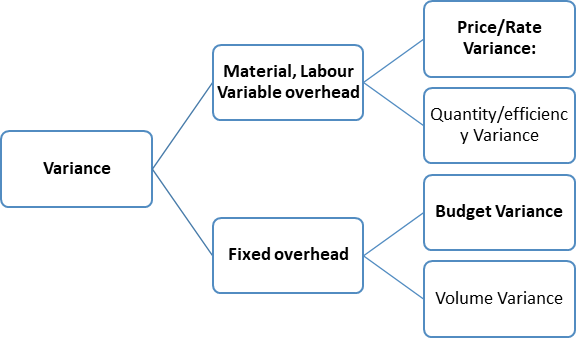# Variance analysis

05/05/2020

Variance analysis is the quantitative investigation of the difference between actual and planned behavior. This analysis is used to maintain control over a business. For example, if you budget for sales to be Rs. 10,000 and actual sales are Rs. 8,000, variance analysis yields a difference of Rs. 2,000. Variance analysis is especially effective when you review the amount of a variance on a trend line, so that sudden changes in the variance level from month to month are more readily apparent. Variance analysis also involves the investigation of these differences, so that the outcome is a statement of the difference from expectations, and an interpretation of why the variance occurred. To continue with the example, a complete analysis of the sales variance would be:

“Sales during the month were Rs. 2,000 lower than the budget of Rs. 10,000. This variance was primarily caused by the loss of ABC customer at the end of the preceding month, which usually buys Rs. 1,800 per month from the company. We lost ABC customer because we had several instances of late deliveries to it over the past few months.”

This level of detailed variance analysis allows management to understand why fluctuations occur in its business, and what it can do to change the situation.Here are the most commonly-derived variances used in variance analysis (they are linked to more complete descriptions, as well as examples):

• Purchase price variance. The actual price paid for materials used in the production process, minus the standard cost, multiplied by the number of units used.
• Labor rate variance. The actual price paid for the direct labor used in the production process, minus its standard cost, multiplied by the number of units used.
• Variable overhead spending variance. Subtract the standard variable overhead cost per unit from the actual cost incurred and multiply the remainder by the total unit quantity of output.
• Fixed overhead spending variance. The total amount by which fixed overhead costs exceed their total standard cost for the reporting period.
• Selling price variance. The actual selling price, minus the standard selling price, multiplied by the number of units sold.
• Material yield variance. Subtract the total standard quantity of materials that are supposed to be used from the actual level of use and multiply the remainder by the standard price per unit.
• Labor efficiency variance. Subtract the standard quantity of labor consumed from the actual amount and multiply the remainder by the standard labor rate per hour.
• Variable overhead efficiency variance. Subtract the budgeted units of activity on which the variable overhead is charged from the actual units of activity, multiplied by the standard variable overhead cost per unit.

It is not necessary to track all of the preceding variances. In many organizations, it may be sufficient to review just one or two variances. For example, a services organization (such as a consulting business) might be solely concerned with the labor efficiency variance, while a manufacturing business in a highly competitive market might be mostly concerned with the purchase price variance. In other words, put most of the variance analysis effort into those variances that make the most difference to the company if the underlying issues can be rectified.

There are several problems with variance analysis that keep many companies from using it. They are:

• Time delay. The accounting staff compiles the variances at the end of the month before issuing the results to the management team. In a fast-paced environment, management needs feedback much faster than once a month, and so tends to rely upon other measurements or warning flags that are generated on the spot (especially in the production area).
• Variance source information. Many of the reasons for variances are not located in the accounting records, so the accounting staff has to sort through such information as bills of material, labor routings, and overtime records to determine the causes of problems. The extra work is only cost-effective when management can actively correct problems based on this information.
• Standard setting. Variance analysis is essentially a comparison of actual results to an arbitrary standard that may have been derived from political bargaining. Consequently, the resulting variance may not yield any useful information.

Many companies prefer to use horizontal analysis, rather than variance analysis, to investigate and interpret their financial results. Under this approach, the results of multiple periods are listed side-by-side, so that trends can be easily discerned.